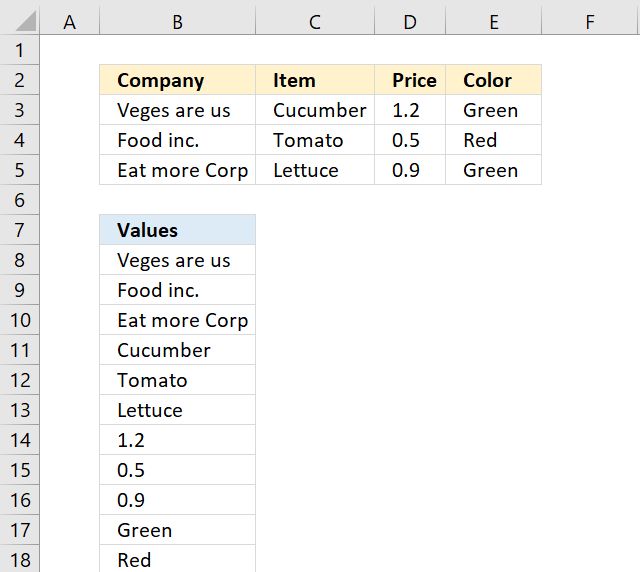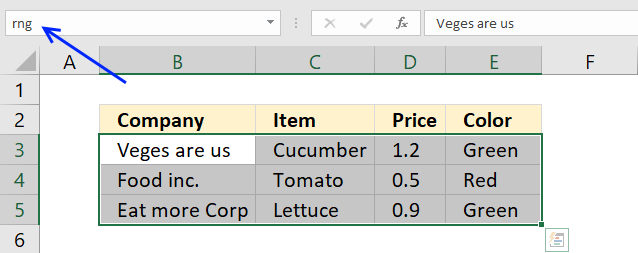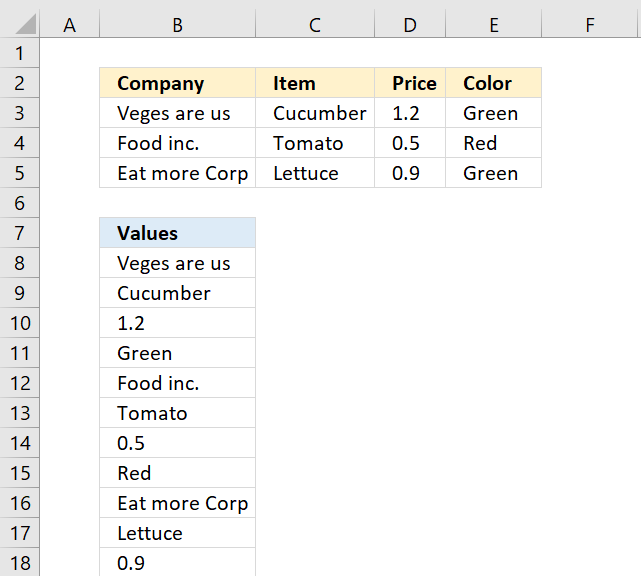Author: Oscar Cronquist Article last updated on February 09, 2018The formula in cell B8 uses a named range to calculate the row and column needed to extract the correct value.

=INDEX(rng, MOD(ROW(A1)-1, ROWS(rng))+1, QUOTIENT(ROW(A1)-1, ROWS(rng))+1)To create a named range simply select the cell range (B3:E5) and click in name box. Type a name for that range, I named the cell range rng. Press Enter, that's it.

### Explaining formula in cell B8

The INDEX function needs a row and column number in order to get the correct value.

The ROWS function returns the number of rows in a cell range.

ROWS(rng) becomes ROWS(B3:E5) and returns 3.

The ROW function returns the row number of a cell reference.

ROW(A1) returns 1.

To calculate the row number I use the MOD function to build a repeating number sequence. There are three rows in B3:E5 so the sequence must be 1,2,3,1,2,3, ... and so on.

MOD(ROW(A1)-1, ROWS(rng))+1

becomes

MOD(1-1, 3)+1

becomes

MOD(0, 3) + 1 and returns 1.

To calculate the column number I use the QUOTIENT function to build a repeating number sequence: 1,1,1,2,2,2,3 ... and so on.

QUOTIENT(ROW(A1)-1, ROWS(rng))+1

becomes

QUOTIENT(1-1, 3)+1

becomes

QUOTIENT(0, 3)+1 and returns 1.

The INDEX function then returns the value in row 1 and column 1 from cell range 3:E5.

INDEX(rng, MOD(ROW(A1)-1, ROWS(rng))+1, QUOTIENT(ROW(A1)-1, ROWS(rng))+1)

becomes

INDEX(rng, 1, 1)

and returns "Veges are us" in cell B8.

### Returning values row by rowThe following formula gets the values row by row:

=INDEX(rng, QUOTIENT(ROW(A1)-1, COLUMNS(rng))+1, MOD(ROW(A1)-1, COLUMNS(rng))+1)

### Download Excel *.xlsx file

Rearrange a cell range to vertically distributed values.xlsx